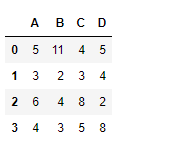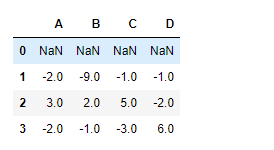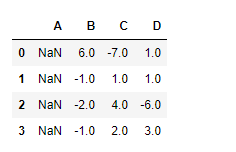# Python | Pandas dataframe.diff()

Python is a great language for doing data analysis, primarily because of the fantastic ecosystem of data-centric python packages. Pandas is one of those packages and makes importing and analyzing data much easier.

Pandas` dataframe.diff()` is used to find the first discrete difference of objects over the given axis. We can provide a period value to shift for forming the difference.

Syntax: DataFrame.diff(periods=1, axis=0)

Parameters:
periods : Periods to shift for forming difference
axis : Take difference over rows (0) or columns (1).

Returns: diffed : DataFrame

Example #1: Use `diff()` function to find the discrete difference over the index axis with period value equal to 1.

 `# importing pandas as pd ` `import` `pandas as pd ` ` `  `# Creating the dataframe ` `df ``=` `pd.DataFrame({``"A"``:[``5``, ``3``, ``6``, ``4``], ` `                   ``"B"``:[``11``, ``2``, ``4``, ``3``],  ` `                   ``"C"``:[``4``, ``3``, ``8``, ``5``], ` `                   ``"D"``:[``5``, ``4``, ``2``, ``8``]}) ` ` `  `# Print the dataframe ` `df `Now find the discrete difference over the index axis.

 `# To find the discrete difference ` `df.diff(axis ``=` `0``, periods ``=` `1``) `

Output :The output is a dataframe with cells containing the discrete difference over the index axis. The value present in each cell is the difference of current cell value with the previous row corresponding cell. Notice, the first row is `NaN` filled. This is because there is no row above that to find the difference with so it is treated as `NaN`.

Example #2: Use `diff()` function to find the discrete difference over the column axis with period value equal to 1.

 `# importing pandas as pd ` `import` `pandas as pd ` ` `  `# Creating the dataframe ` `df ``=` `pd.DataFrame({``"A"``:[``5``, ``3``, ``6``, ``4``], ` `                   ``"B"``:[``11``, ``2``, ``4``, ``3``],  ` `                   ``"C"``:[``4``, ``3``, ``8``, ``5``],  ` `                   ``"D"``:[``5``, ``4``, ``2``, ``8``]}) ` ` `  `# To find the discrete difference ` `df.diff(axis ``=` `1``, periods ``=` `1``) `

Output :The output is a dataframe with cells containing the discrete difference over the column axis. The value present in each cell is the difference of current cell value with the previous column corresponding cell. Notice, the first column is `NaN` filled. This is because there is no column to the left of it to find the difference with so it is treated as `NaN`.

Attention geek! Strengthen your foundations with the Python Programming Foundation Course and learn the basics.

To begin with, your interview preparations Enhance your Data Structures concepts with the Python DS Course.

My Personal Notes arrow_drop_upCheck out this Author's contributed articles.

If you like GeeksforGeeks and would like to contribute, you can also write an article using contribute.geeksforgeeks.org or mail your article to contribute@geeksforgeeks.org. See your article appearing on the GeeksforGeeks main page and help other Geeks.

Please Improve this article if you find anything incorrect by clicking on the "Improve Article" button below.

Article Tags :

1

Please write to us at contribute@geeksforgeeks.org to report any issue with the above content.